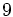# Dihedral group:D18

View a complete list of particular groups (this is a very huge list!)[SHOW MORE]

## Definition

The group is defined as the dihedral group of order math>18[/itex] and degree$9$. In other words, it has the presentation:$\langle a,x \mid a^9 = x^2 = e, xax = a^{-1} \rangle$.

## GAP implementation

### Group ID

This finite group has order 18 and has ID 1 among the groups of order 18 in GAP's SmallGroup library. For context, there are groups of order 18. It can thus be defined using GAP's SmallGroup function as:

SmallGroup(18,1)

For instance, we can use the following assignment in GAP to create the group and name it$G$:

gap> G := SmallGroup(18,1);

Conversely, to check whether a given group$G$ is in fact the group we want, we can use GAP's IdGroup function:

IdGroup(G) = [18,1]

or just do:

IdGroup(G)

to have GAP output the group ID, that we can then compare to what we want.

### Other descriptions

The group can be constructed using GAP's DihedralGroup function:

DihedralGroup(18)